#### 2.2.2Hooke’s Law

The elastic behavior of solids can be modeled by the same principle which governs spring deformations, known as Hooke’s law. The law suggests a linear relationship between the deformation and the force applied to a string or in a solid. Hence, the relation stress-strain in solid bodies in the elastic regime can be expressed by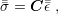(2.13)

where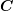is a linear mapping between the two tensors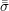and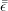. This implies thatis a fourth-order tensor with 81 components (each of the 9 strain tensor components is related with each of the 9 stress tensor components). However, due to the symmetry of the strain and stress tensors the number of independent components ofcan be reduced to 21. This simplification process can be seen graphically in Fig. 2.8. Therefore a 6x6 symmetric matrix is a convenient way for representing the tensorand the stress-strain relation can be rewritten as in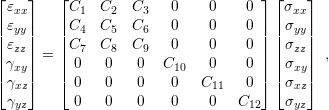(2.14)

where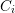are constants to be determined in the next sections. The matrix in (2.14) is also known as stiffness matrix.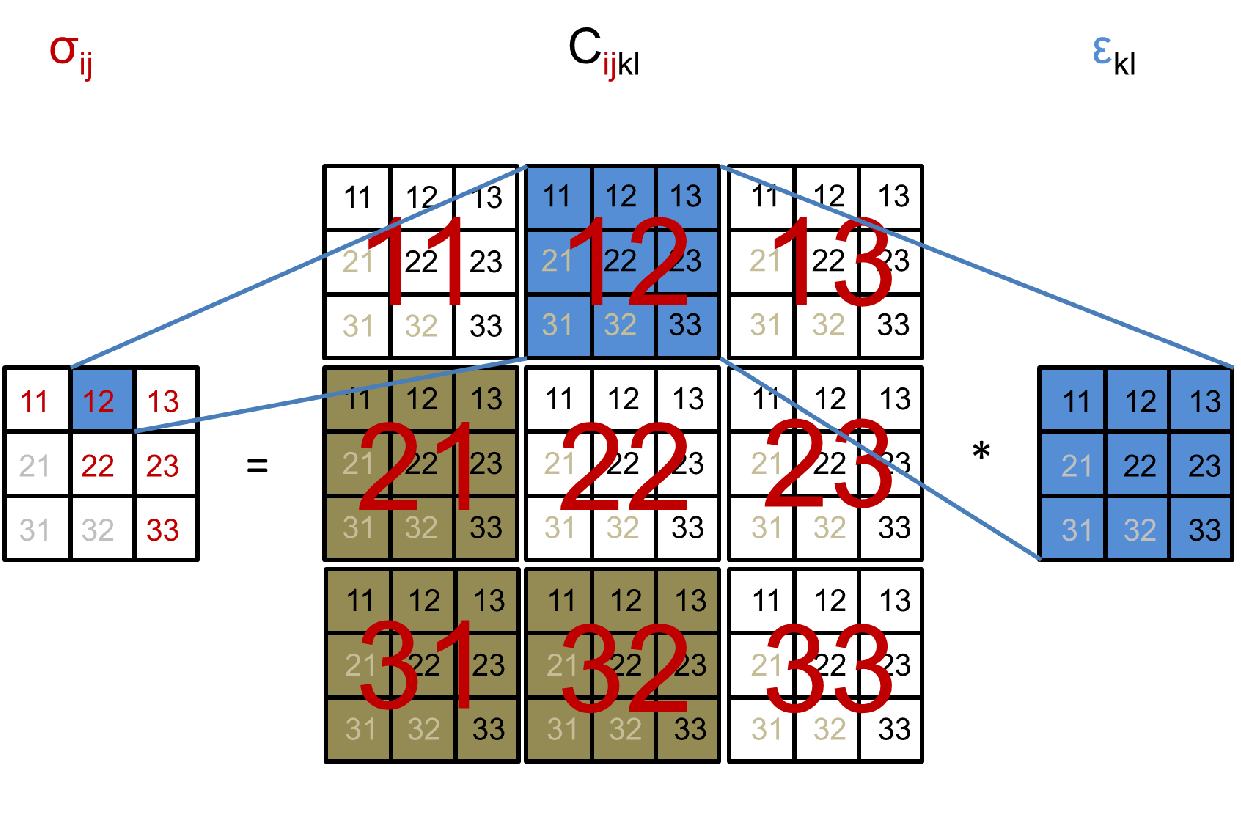Figure 2.8.: Visualization of the tensorial Hooke’s law. Strain symmetry forces symmetry on thetensor on all components in the form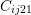,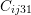, and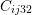indicated by numbers in gray. Strain symmetry is enforced on the components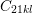,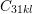, and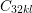indicated by the brown squares.

##### Coefficient Determination for the Normal Components

The coefficients of the stiffness matrix for the normal components can be obtained from the superposition of two effects in a solid. For doing so, a force which is applied to a body is considered, as depicted in Fig. 2.9.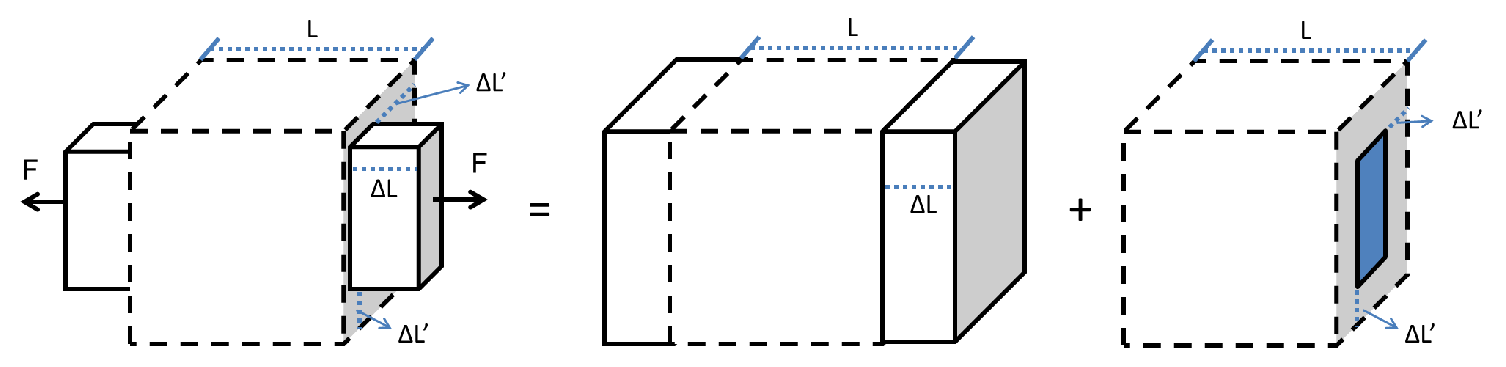Figure 2.9.: Superposition of effects during an uniaxial loading of a body, where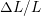is the normal strain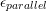along the direction of the applied force and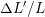is the normal strain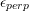in the directions perpendicular to the force.

The body stretches in the same direction of the load and shrinks in the perpendicular direction. These two deformations can be treated separately and then added together by the principle of linear superposition of the resulting strains produced by each phenomena depicted in Fig. 2.9. The strain in the parallel direction is given by the uniaxial (1D) Hooke’s Law. Consequently, the linear mapping between the stress and strain is described by a constant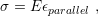(2.15)

where,is the strain in the direction to the parallel to the applied force. It should be noted that in the one-dimensional case the stress and the strain are no longer a tensor but a scalar. The proportionality constant E is known as Young modulus (or elastic modulus). The Young’s modulus depends on the material of the solid and characterizes the material stiffness. Materials with high values of E are stiffer and harder to deform. Typical values of Young’s modulus for different materials essential in semiconductor industry are listed in Table 2.1.

 Table 2.1.: Mechanical properties of common materials used in semiconductor device manufacturing.
 Material Young Modulus (GPa) Poisson ration CTE (K/10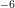) Reference W 410 0.30 4  Cu 124 0.34 16  Al 69-79 0.35 22  Si 107 0.22 5  SiO2 94 0.16 0.5  Ti 116 0.30 9  TiN 440-640 0.25-0.29 9.4  Ta 185 0.35 6.5  SiN 270-320 0.22 3 

The strain in the perpendicular direction is also defined by the uniaxial Hooke’s Law but with a different proportionality constant as in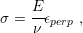(2.16)

whereis known as the Poisson ratio and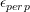is the strain in a direction perpendicular to the applied force. The Poisson ration describes the deformation in the perpendicular direction as a proportion of the deformation in the parallel direction. Typical values of the Poisson ratio for semiconductor materials are also given in Table 2.1.

For a general treatment, forces applied in all three coordinate directions should be considered. A complete description for a 3D system is given by the set of equations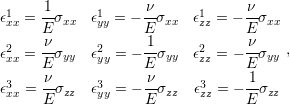(2.17)

where the indexes xx, yy, and zz stand for the normal strains and stresses. The super-indexes 1, 2, and 3 refer to the deformations induced by forces in the x, y, and z directions, respectively.

Finally, all the strains in each direction can be superimposed (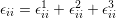) and with some algebraic manipulation the final total strain in each direction is given by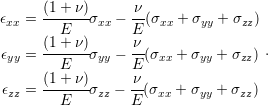(2.18)

##### Coefficient Determination for the Shear Components

Regarding the shear components, the coefficient determination follows directly from the uniaxial Hooke’s Law. Similarly to the previous case, the shear stress relates to the strain by a coefficient as in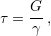(2.19)

whereand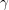are the shear stress and strain. G is the shear modulus and defines the resistance of a body to torsion. The shear modulus can be written in terms of the Young modulus and the Poisson ratio according to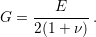(2.20)

In conclusion, Hooke’s law with all constants is given by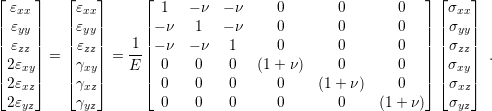(2.21)

The inverse relation with the stress on the left side is written as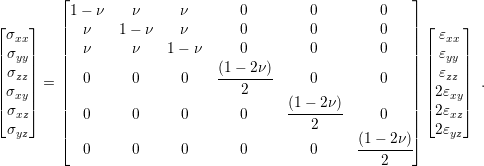(2.22)